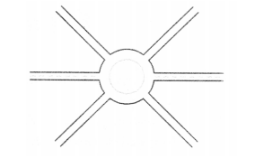# A boy is moving on a straight road against`
Question:

A boy is moving on a straight road against a frictional force of $5 \mathrm{~N}$. After travelling a distance of $1.5 \mathrm{~km}$ he forgot the correct path at a round about (Fig. 1) of radius $100 \mathrm{~m}$.Solution:

However, he moves on the circular path for one and half cycle and then he moves forward upto $2.0 \mathrm{~km}$.

Calculate the work done by him.

Here, $F=5 \mathrm{~N}$, Distance travelled $S=1500+3 \pi r+2000=4442.86 m$

$\therefore W=F \times S=5 \times 4442.86=22214.3 \mathrm{~J}$.